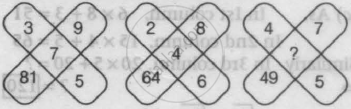# Find Out the Missing Number. - Logical Reasoning

MCQ

Find out the missing number.• 1

• 8

• 6

• 16

#### Solution

6
Explanation:
In the first figure,
81/9-(5-3)=9-2=7   ...(middle number)

In the second figure,
64/8-(6-2)=8-4=4  ...(middle number)

Similarly, in the third figure,
49/7-(5-4)=7-1= 6   ....(middle number)

Concept: Inserting the Missing Character (Entrance Exam)
Is there an error in this question or solution?